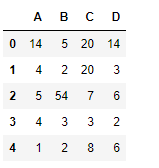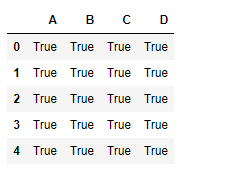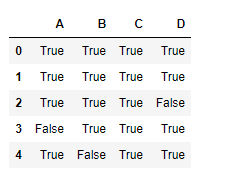# Python | Pandas dataframe.notna()

• Difficulty Level : Medium
• Last Updated : 27 Aug, 2021

Python is a great language for doing data analysis, primarily because of the fantastic ecosystem of data-centric python packages. Pandas is one of those packages and makes importing and analyzing data much easier.
Pandas dataframe.notna() function detects existing/ non-missing values in the dataframe. The function returns a boolean object having the same size as that of the object on which it is applied, indicating whether each individual value is a na value or not. All of the non-missing values gets mapped to true and missing values get mapped to false.
Note : Characters such as empty strings ” or numpy.inf are not considered NA values. (unless you set pandas.options.mode.use_inf_as_na = True).

Syntax: DataFrame.notna()
Returns : Mask of bool values for each element in DataFrame that indicates whether an element is not an NA value

Example #1: Use notna() function to find all the non-missing value in the dataframe.

## Python3

 `# importing pandas as pd``import` `pandas as pd` `# Creating the first dataframe``df ``=` `pd.DataFrame({``"A"``:[``14``, ``4``, ``5``, ``4``, ``1``],``                   ``"B"``:[``5``, ``2``, ``54``, ``3``, ``2``],``                   ``"C"``:[``20``, ``20``, ``7``, ``3``, ``8``],``                   ``"D"``:[``14``, ``3``, ``6``, ``2``, ``6``]})` `# Print the dataframe``df`Let’s use the dataframe.notna() function to find all the non-missing values in the dataframe.

## Python3

 `# find non-na values``df.notna()`

Output :As we can see in the output, all the non-missing values in the dataframe has been mapped to true. There is no false value as there is no missing value in the dataframe.

Example #2: Use notna() function to find the non-missing values, when there are missing values in the dataframe.

## Python3

 `# importing pandas as pd``import` `pandas as pd` `# Creating the dataframe``df ``=` `pd.DataFrame({``"A"``:[``12``, ``4``, ``5``, ``None``, ``1``],``                   ``"B"``:[``7``, ``2``, ``54``, ``3``, ``None``],``                   ``"C"``:[``20``, ``16``, ``11``, ``3``, ``8``],``                   ``"D"``:[``14``, ``3``, ``None``, ``2``, ``6``]})` `# find non-missing values``df.notna()`

Output :As we can see in the output, cells which were having na values were mapped as false and all the cells which were having non-missing values were mapped as true.

My Personal Notes arrow_drop_up